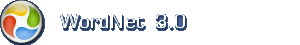# English dictionary

Hint: Wildcards can be used multiple times in a query.

## English noun: computation

1. computation (act) the procedure of calculating; determining something by mathematical or logical methods

Synonymscalculation, computing

Narrower (hyponym)mathematical operation, mathematical process, number crunching, operation, recalculation, transposition

2. computation (cognition) problem solving that involves numbers or quantities

Synonymscalculation, figuring, reckoning

Narrower (hyponym)approximation, conversion, derivative, derived function, differential, differential coefficient, estimate, estimation, extrapolation, first derivative, idea, integral, interpolation